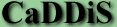BAYESIAN BELIEF NETWORKSA Bayesian Network for a knowledge domain (e.g. a list of diseases and clinical signs) is an encoding of the joint probability density for all of the variables which are included in the list.

Given a list of diseases and signs, and a sufficient understanding of the inter-relationships between these factors, it would be possible for our model of the system to be summarised mathematically by a large list of probabilities of the form

P(Anaplasmosis=TRUE, Anthrax=FALSE,... T.B.=FALSE, Abortion=TRUE, Anaemia=TRUE, Change in Urine=FALSE,.... Wasting=FALSE)=0.0003

P(Anaplasmosis=TRUE, Anthrax=FALSE,... T.B.=FALSE, Abortion=FALSE, Anaemia=TRUE, Change in Urine=FALSE,.... Wasting=FALSE)=0.007

P(Anaplasmosis=FALSE, Anthrax=TRUE,... T.B.=FALSE, Abortion=TRUE, Anaemia=TRUE, Change in Urine=FALSE,.... Wasting=TRUE)=0.00002

and so on....

In the case of CaDDiS, where we consider 20 diseases and 27 clinical signs, we would require 2 to the power of 47 different probabilities to define the joint probability distribution. The generation of these 140 trillion probabilities would not be a trivial exercise! It is easier to define our knowledge of the system in terms of conditional probabilities, but the derivation of the required joint p.d.f. from conditionals is still computationally prohibitive. The Bayesian Network uses the concept of Conditional Independence to simplify our calculations, allowing us to derive the information which we need from the joint distribution using a much smaller number of conditional probabilities. The system is defined in terms of probabilities such as

P(Anaemia | Anaplasmosis)="the probability of a cow exhibiting anaemia given that it is infected with anaplasmosis" or

P(Anthrax | Constipation, Dyspnoea)= " the probability of a cow being infected with anaemia given that it is exhibiting constipation and dyspnoea".

These equations can be simplified using conditional independence where, for example

P( FMD | Lameness and foot lesions)=P(FMD | foot lesions) (the knowledge that the animal is lame adds nothing to our understanding if we already know that the cow has foot lesions),

or

P(Anthrax | Tongue lesions) = P(Anthrax) (tongue lesions have nothing to do with anthrax.. their presence should not directly affect our estimate of whether a cow has that disease.

The effect of these conditional probabilities can be summarised graphically. Each disease or sign is represented as a point, and two points A and B are only connected by lines if there are no other events C(i) such that

P(A | B, C(1), C(2),... C(n)) = P(A | C(1), C(2),... C(n)),

i.e. that A and B are never conditionally independent. The resulting graph is known as a network.

Where a clinical sign is observed in an animal, that variable in the network is specified: e.g., Coughing=TRUE is given a belief of 1. This information will affect the conditional probabilities of those other events which the Coughing node has lines into:

e.g. where P(ECF | coughing) is a valid conditional probability, the observation of coughing will affect our belief in the presence of ECF in the cow.

The effects of new information are propagated through the network using Bayes's Theorem, changing the belief values of other diseases and signs. Hence, the graph is a Bayesian Belief Network.

It is possible to break the overall graph down into smaller sub-sets within which information flows are largely self-contained. This approach allows the propagation of information to proceed much more efficiently. More details of this topic can be found in Lauritzen and Spiegelhalter (1988).